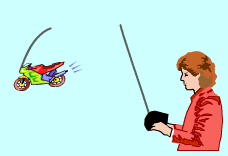Ch 4. The Mean Value Theorem Multimedia Engineering Math Maximum & Minimum Rolle'sTheorem Mean ValueTheorem MonotonicFunctions First DerivativeTest Concavity &Inflection SecondDerivative Test
 Chapter 1. Limits 2. Derivatives I 3. Derivatives II 4. Mean Value 5. Curve Sketching 6. Integrals 7. Inverse Functions 8. Integration Tech. 9. Integrate App. 10. Parametric Eqs. 11. Polar Coord. 12. Series Appendix Basic Math Units Search eBooks Dynamics Fluids Math Mechanics Statics Thermodynamics Author(s): Hengzhong Wen Chean Chin Ngo Meirong Huang Kurt Gramoll ©Kurt GramollMATHEMATICS - CASE STUDY IntroductionRemote Control Motorcycle In order to improve her ability to control and operate a remote control car, Judy has decided to analyze the car data, such as velocity, acceleration, weight, fraction, power, and energy. To start the analysis, she examines a basic diagram of the displacement curve of the motorcycle during its operation. What is known: The operation lasts 30 seconds. From 0 to 10 seconds and 22 to 30 seconds, the acceleration is positive. From 10 to 22 seconds, the acceleration is negative. The velocity is 0 at 7, 14 and 26 seconds. The velocity changes from positive to negative at 7 and 26 seconds. The velocity changes from negative to positive at 14 seconds. Questions Plot the rough displacement curve. Approach Use the Test for Concavity to find the concavity of the function The inflection point is the point where the curve changes from concave upward to concave downward or from concave downward to concave upward. Velocity is the first derivative of displacement with respect to time. Acceleration is the second derivative of displacement with respect to time.